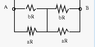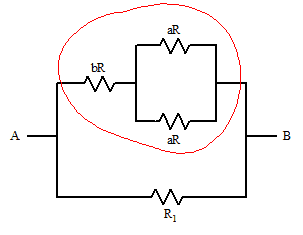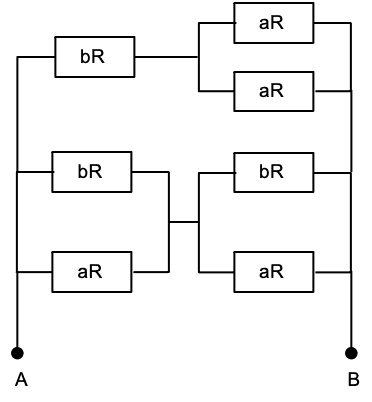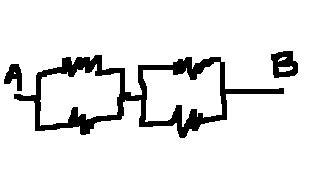# Understanding Complex Circuit Configurations

• JoeyBob

#### JoeyBob

Homework Statement
Find the equivalent resistance (see image)
Relevant Equations
1/r = 1/r+1/r
r=r+r
Having more difficulty understanding the concept, thus I am not showing values.

What is causing me confusion is the line in the middle. The first aR and bR are obviously in parallel, but the second aR and bR confuse me. I tried calculating the equivalent resistance from the first aR and bR and then add the equivalent resistance from the second two, but that gave a number way too high. Treating the whole circuit as parallel also gives the wrong answer.

#### Attachments

•Circuit.PNG
807 bytes · Views: 80

Maybe this tutorial will help on Kirchoff's laws:

https://www.electronics-tutorials.ws/dccircuits/dcp_4.html

Maybe this tutorial will help on Kirchoff's laws:

https://www.electronics-tutorials.ws/dccircuits/dcp_4.html

I don't think this question really relates that much to kirchhoffs laws. Its strictly about finding the total resistance, it has nothing to do with solving for resitance using current and voltage. The resistance values for each resistor are given, its a question of knowing how to add them up.

I can say that the resistance of bR is 34.04 and aR is 44.16. The answer to the question is 22.82, but I don't know the steps to get there.

Looks to me like the given answer may be incorrect. This happens sometimes when problems are "refreshed" by the publisher and answer is not updated.

Where did the values of aR and bR come from, a previous question step in a larger problem?

Looks to me like the given answer may be incorrect. This happens sometimes when problems are "refreshed" by the publisher and answer is not updated.

Where did the values of aR and bR come from, a previous question step in a larger problem?

Its a distinct question. Ill just attach the whole thing here.

#### Attachments

Its a distinct question. Ill just attach the whole thing here.
This is a basic series/parallel problem. Nothing fancy is needed but you need to understand what series and parallel mean and how to use the formulae.

I’ve just done it and got the official answer of 22.82 Ω . Though I would point out this should be rounded to 23 Ω to match the significant figures in the data. Also the original question says ‘Ohms’ which is wrong; it should be written using lower case: ‘ohms’.

Imagine shortening the vertical wire (in the middle of the bottom two rows of the circuit) to a point (P). This has no effect on the currents/voltages in the rest of the circuit.

It should now be clear you have [aR and bR in parallel] on the left of P and [aR and bR in parallel] on the right of P.

These two parallel pairs are in series.

Does that help?

•Delta2
I agree with the answer given 22.815Ω.

The way to solve problems like this is to go through some sequential steps to reduce the number of resistors. So, in the original circuit diagram you will use the formulas for resistors in series or parallel to find an equivalent resistor to replace each pair of resistors that are either in parallel or in series. Then you can redraw the schematic with fewer equivalent resistors and repeat the process until you only have one resistor left.

Its a distinct question. Ill just attach the whole thing here.
...and this is why it's important to post the full question as it was given. Context is everything.

This is a basic series/parallel problem. Nothing fancy is needed but you need to understand what series and parallel mean and how to use the formulae.

I’ve just done it and got the official answer of 22.82 Ω . Though I would point out this should be rounded to 23 Ω to match the significant figures in the data. Also the original question says ‘Ohms’ which is wrong; it should be written using lower case: ‘ohms’.

Imagine shortening the vertical wire (in the middle of the bottom two rows of the circuit) to a point (P). This has no effect on the currents/voltages in the rest of the circuit.

It should now be clear you have [aR and bR in parallel] on the left of P and [aR and bR in parallel] on the right of P.

These two parallel pairs are in series.

Does that help?

I thought that's what I was trying.
See work attached. I treat them both as parallels then add them.

#### Attachments

•Delta2
I thought that's what I was trying.
See work attached. I treat them both as parallels then add them.
That's good, so far. But, there are other resistors too; you've now reduced the circuit from 7 to 4 resistors.

•Delta2
That's good, so far. But, there are other resistors too; you've now reduced the circuit from 7 to 4 resistors.
Sure but the other resistors arent between A and B. Furthermore my answer is already too big, adding more resistors would only make it bigger.

Sure but the other resistors arent between A and B.
You can't draw a path starting at A and going through those "other" resistors to B?

You can't draw a path starting at A and going through those "other" resistors to B?
For some reason thatws where I thought the battery was. I guess that's how you do it, though I would argue no current/voltage (theres no battery) would mean there is also no resistance.

For some reason thatws where I thought the battery was. I guess that's how you do it, though I would argue no current/voltage (theres no battery) would mean there is also no resistance.
Resistance is a property of materials which exists whether you measure it or not; similar to my weight, which doesn't go away when I step off the scale in my bathroom. If it makes you feel better you can draw in a battery between points A and B.

•Delta2
What value did you get for the net resistance of the bottom four resistors? Let's call that R1. Then your circuit is reduced to:and you'll now want to move your attention to the upper branch circled in red. Reduce that to a single resistance value. What will the resulting "reduced" circuit look like then?

•Delta2
Sure but the other resistors arent between A and B.
Do you see why the circuit below has the same equivalent resistance between A and B as the original circuit?•Delta2
Resistance is a property of materials which exists whether you measure it or not; similar to my weight, which doesn't go away when I step off the scale in my bathroom. If it makes you feel better you can draw in a battery between points A and B.

But V=IR, so if there's no voltage at a resistor (V=0), doesn't that mean it offers no resistance (0/I=R=0)?

Doesn't resistance change on a resistor when different voltages are applied?

both ends of the line in the middle represent the same point, because they are at the same potential.
you have a pair of two parallel resistors.•Delta2
But V=IR, so if there's no voltage at a resistor (V=0), doesn't that mean it offers no resistance (0/I=R=0)?

Doesn't resistance change on a resistor when different voltages are applied?
No. Normal resistors don't change their resistance. In your example if you have V=0 then you will also have I=0. In this case some versions of ohms law, like R=V/I=0/0 don't make sense. Other versions like V=I⋅R ⇒ 0=0⋅R do make sense.

But V=IR, so if there's no voltage at a resistor (V=0), doesn't that mean it offers no resistance (0/I=R=0)?
No. Current is zero in this case. R = V/I = 0/0 which is not zero, it is undefined. This simply means you can't calculate resistance from values of voltage and current when they are both zero.

If it helps, just imagine a battery is going to be connected to A and B later. You are working out the resistance of the circuit in advance.

Doesn't resistance change on a resistor when different voltages are applied?
No. Try this:
You have a 3Ω resistor.
a) You apply 12V to the esistor; what current flows?
b) You apply 24V to the resistor; what current flows?
What is the resistance in each case?

(There are situations where the resistance of an object changes with voltage, for example if the resulting current makes the temperature increase. But that's not relevant in this question.)

•Delta2
But V=IR, so if there's no voltage at a resistor (V=0), doesn't that mean it offers no resistance (0/I=R=0)?

Doesn't resistance change on a resistor when different voltages are applied?
Inertial mass is thought of by some as an object's resistance to change in its state of motion when an unbalanced force acts on it. This doesn't mean that an object is massless if no unbalanced force acts on it.

No. Current is zero in this case. R = V/I = 0/0 which is not zero, it is undefined. This simply means you can't calculate resistance from values of voltage and current when they are both zero.

If it helps, just imagine a battery is going to be connected to A and B later. You are working out the resistance of the circuit in advance.

No. Try this:
You have a 3Ω resistor.
a) You apply 12V to the esistor; what current flows?
b) You apply 24V to the resistor; what current flows?
What is the resistance in each case?

(There are situations where the resistance of an object changes with voltage, for example if the resulting current makes the temperature increase. But that's not relevant in this question.)

I see, so when current changes voltage will change to ensure the resistance is constant.

Is that why circuits with no resistors heat up?

I see, so when current changes voltage will change to ensure the resistance is constant.
I wouldn't say it that way. The value of resistance is determined by the size, shape and material the resistor is made from (and also the temperature of the material). Resistance doesn't change unless the size, shape or material (or temperature) change.

It is a property of (simple resistors) that the current is proportional to the applied voltage. For example, tripling the voltage across a resistor will triple the current through it. I highly recommend reading about Ohm's law in your textbook and notes. There are some good YouTube videos too. It is important to understand Ohm's law.
Is that why circuits with no resistors heat up?
I don't know if you are joking. Can you give an example of a circuit with no resistors that heats up?

I wouldn't say it that way. The value of resistance is determined by the size, shape and material the resistor is made from (and also the temperature of the material). Resistance doesn't change unless the size, shape or material (or temperature) change.

It is a property of (simple resistors) that the current is proportional to the applied voltage. For example, tripling the voltage across a resistor will triple the current through it. I highly recommend reading about Ohm's law in your textbook and notes. There are some good YouTube videos too. It is important to understand Ohm's law.

I don't know if you are joking. Can you give an example of a circuit with no resistors that heats up?

Like during a short circuit, the wire heats up. There's no real resistor present (besides the resistance from the wire).

Like during a short circuit, the wire heats up. There's no real resistor present (besides the resistance from the wire).
A wire causing a short-circuit has a small (but not zero) resistance. Because the resistance is so small, the current is very large (Remember I = V/R). The thermal power produced is P = I²R. Note that P depends on current squared: when R is small, I is large and I² is even larger.

If you short-circuit a supply such as a battery, the battery gets hot. That's because the battery has some resistance of its own (called internal resistance) due to the materials it is made from. When short-circuited an abnomally large current flows through the battery and wire. Heating occurs in the wire because of the wire's resistance. And heating occurs in the battery because of the battery's internal resistance.

Whenever electrical heating occur, it is caused by current flowing through a resistance.

(The only things with no resistance are superconductors, which don't concern us here.)